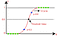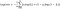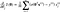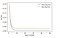# Logistic Regression Using SGD from Scratch

While Python’s Scikit-learn library provides the easy-to-use and efficient SGDClassifier , the objective of this post is to create an own implementation using without using sklearn. Implementing basic models is a great idea to improve your comprehension about how they work.

# Data set

Create a custom dataset using make_classification inbuilt function from sklearn.

# Logistic Regression

Input values (x) are combined linearly using weights or coefficient values to predict an output value (y). A key difference from linear regression is that the output value being modeled is a binary values (0 or 1) rather than a numeric value. To generate the binary values 0 or 1 , here we use sigmoid function.Fig 1. Logistic function

# Loss function

Log Loss is the most important classification metric based on probabilities. For any given problem, a lower log-loss value means better predictions. Log Loss is a slight twist on something called the Likelihood Function. In fact, Log Loss is -1 * the log of the likelihood function.Fig 2. Log loss

# SGD classifier

SGD is a optimization method, SGD Classifier implements regularized linear models with Stochastic Gradient Descent. Stochastic gradient descent considers only 1 random point ( batch size=1 )while changing weights. Logistic Regression by default uses Gradient Descent and as such it would be better to use SGD Classifier on larger data sets ( 50000 entries ).

# Gradient descent

Our goal is to minimize the loss function and to minimize the loss function we have to increasing/decreasing the weights, i.e. fitting them. That can be achieved by the derivative of the loss function with respect to each weight. Derivatives of weights gives us clear picture how loss changes with parameters.Fig 3. Partial derivativeFig 4. Train log loss vs Test log loss
`0.95221333333333340.95`

A Machine learning Enthusiast!

## More from Satishkumar Moparthi

A Machine learning Enthusiast!